Question

During optimal conditions, the rate of change of the population of a certain organism is proportional to the population at time t, in hours. At time t = 0 hours, the population is 300. At time t = 24 hours, the population is 1000. At what time t is the population 500?
(A) t = 2112
(B) t = 24 In 500 In 1000
(D) t = 300e (9)

1.The population is of 500 after 10.22 hours.

Step-by-step explanation:

The rate of change of the population of a certain organism is proportional to the population at time t, in hours.

This means that the population can be modeled by the following differential equation: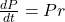In which r is the growth rate.

Solving by separation of variables, then integrating both sides, we have that: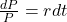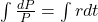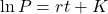Applying the exponential to both sides: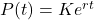In which K is the initial population.

At time t = 0 hours, the population is 300.

This means that K = 300. So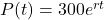At time t = 24 hours, the population is 1000.

This means that P(24) = 1000. We use this to find the growth rate. So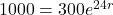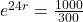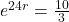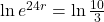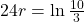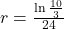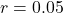So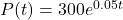At what time t is the population 500?

This is t for which P(t) = 500. So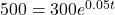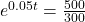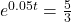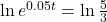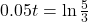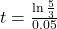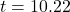The population is of 500 after 10.22 hours.